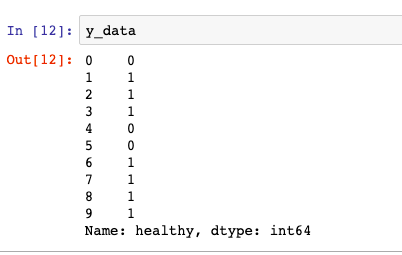Search
•Magdalena Konkiewicz

# Splitting your data to fit any machine learning modelIntroduction

After you have performed data cleaning, data visualizations, and learned details about your data it is time to fit the first machine learning model into it. Today I want to share with you a few very simple lines of code that will divide any data set into variables that you can pass to any machine learning model and start training it.

It is trivially simple but an understanding of how the split function for training and test data sets work is crucial for any Data Scientist. Let’s dive into it.

Preparing data

We are going to create a simple medical data set for visualization purposes. Imagine that we are trying to predict if the patient is healthy or not based on his weight, height, and information if he drinks alcohol or not. Therefore we will have three columns with this information and a fourth column that holds the record if the patient is healthy or not. This is our target variable, something we want to predict. We will have only 10 patients for simplicity here. Let’s create this data frame:

```
import pandas as pd
import numpy as np
client_dictionary = {'weight': [112, 123, 176, 145, 198, 211, 145, 181, 90, 101],
'height': [181, 165, 167, 154, 181, 202, 201, 153, 142, 169],
'drinks alcohol': [0, 1, 1, 1, 0, 1, 1, 1, 0, 1],
'healthy': [0, 1, 1, 1, 0, 0, 1, 1, 1, 1],}
df = pd.DataFrame(client_dictionary)

```

Our data frame looks like this:Separating features from the target variable

We should start with separating features for our model from the target variable. Notice that in our case all columns except ‘healthy’ are features that we want to use for the model. Our target variable is ‘healthy’. We can use the following code to do target separation.

```
x_data = df[['weight', 'height', 'drinks alcohol']]
y_data = df['healthy']

```

Now our x_data looks like this:And y_data looks like this:Using train_test_split

Let’s now use train_test_split from the function from scikit-learn to divide features data (x_data) and target data (y_data) even further into train and test.

```
from sklearn.model_selection import train_test_split
x_train, x_test, y_train, y_test = train_test_split(x_data, y_data ,test_size = 0.2, shuffle=False)

```

As a result, we will have our initial data frame partitioned into four different data sets as pictured below:When calling train_test_split function I have used parameter shuffle=False to achieve the results you can see in the picture above. The first 80 % of the data was assigned to training and the remaining 20% was assigned to test. The default parameter for shuffle is True and this is what you would normally use in a real-life example. This means that the rows before the data is split into test and train are shuffled so their order is changed.

Controlling test-train split fraction

You can control the train_test split fraction by using the test_size parameter. Note that we had it set to 0.2 in our example. It can be any number between 0.0 and 1.0. You do not have to specify the fraction for the train set as by the default it will use all the remaining data that is not taken for the test set.

Train your machine learning model

The data that you have prepared is now ready to be fed to the machine learning model. Let’s just illustrate it with a very simple linear regression model.

```
from sklearn import linear_model
linear_regression_model = linear_model.LinearRegression()
linear_regression_model.fit(x_train, y_train)

```

The model above is trained using x_train and y_train samples. You can now make predictions on the test set using the following code.

```
y_pred = linear_regression_model.predict(x_test)

```

Now if you would like to assess how good your model is you would need to compare your predictions on the test set (y_pred) with the real target values for the test set (y_test). But this is a different story and we will not cover this here.

Conclusion

You have learned how to separate your data set into features and target variable, and then further split it into test and train parts. And all of this just with few lines of code, elegant and simple.

Furthermore, I hope you got to understand how the split works and you will be using it wisely from now on instead of just copy-pasting the code you have found.

And if you do copy-paste code from time to time, it is still ok, but what satisfaction you have where you actually get to understand it.